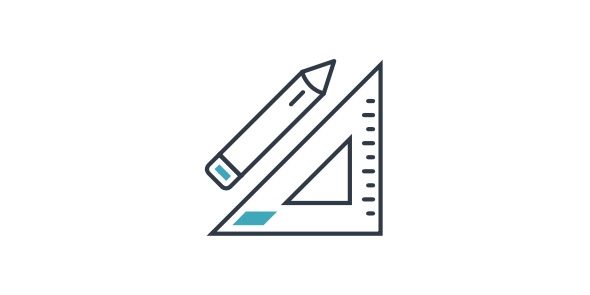# Amazing Quiz On 3rd Grade Math

15 Questions | Total Attempts: 245Settings• 1.
What is 8 divided by 2?
• A.

4

• B.

28

• C.

6

• D.

82

• 2.
What is 317 rounded to the nearest 100?
• A.

200

• B.

400

• C.

500

• D.

300

• 3.
What number must be multiplied by 6 to get 54?
• A.

7

• B.

9

• C.

8

• D.

6

• 4.
What is 26 + 17?
• A.

23

• B.

53

• C.

43

• D.

33

• 5.
What is 3291 rounded to the nearest 1000?
• A.

4000

• B.

3000

• C.

5000

• D.

3500

• 6.
What is 1 x 4?
• A.

4

• B.

4-Jan

• C.

0

• D.

1

• 7.
What is 17 rounded to the nearest 10?
• A.

30

• B.

20

• C.

10

• D.

40

• 8.
If Sarah had 8 cookies and gave 5 to Emma, how many cookies would Sarah have left?
• A.

11

• B.

5

• C.

3

• D.

8

• 9.
How many millimeters are in a meter?
• A.

10

• B.

100

• C.

1

• D.

1000

• 10.
If you practice basketball for 1 hour each day for 4 days, how many total hours do you practice?
• A.

8

• B.

16

• C.

4

• D.

1

• 11.
What unit would you use to measure your weight?
• A.

Ounces

• B.

Feet

• C.

Pounds

• D.

Inches

• 12.
What is the perimeter of a square whose sides are 4 inches each?
• A.

8 Inches

• B.

16 Square Inches

• C.

16 Inches

• D.

64 Inches

• 13.
What symbol means "subtract"?
• A.

X

• B.

-

• C.

+

• D.

=

• 14.
How much is 1 quarter, 1 dime, and 3 pennies?
• A.

37 Cents

• B.

39 Cents

• C.

38 Cents

• D.

35 Cents

• 15.
What is (2/6 - 0) in lowest terms?
• A.

0

• B.

6-Feb

• C.

6-Mar

• D.

3-Jan

Related Topics